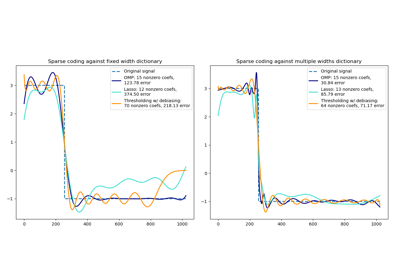# sklearn.decomposition.SparseCoder¶

class sklearn.decomposition.SparseCoder(dictionary, *, transform_algorithm='omp', transform_n_nonzero_coefs=None, transform_alpha=None, split_sign=False, n_jobs=None, positive_code=False, transform_max_iter=1000)[source]

Sparse coding.

Finds a sparse representation of data against a fixed, precomputed dictionary.

Each row of the result is the solution to a sparse coding problem. The goal is to find a sparse array code such that:

X ~= code * dictionary


Read more in the User Guide.

Parameters:
dictionaryndarray of shape (n_components, n_features)

The dictionary atoms used for sparse coding. Lines are assumed to be normalized to unit norm.

transform_algorithm{‘lasso_lars’, ‘lasso_cd’, ‘lars’, ‘omp’, ‘threshold’}, default=’omp’

Algorithm used to transform the data:

• 'lars': uses the least angle regression method (linear_model.lars_path);

• 'lasso_lars': uses Lars to compute the Lasso solution;

• 'lasso_cd': uses the coordinate descent method to compute the Lasso solution (linear_model.Lasso). 'lasso_lars' will be faster if the estimated components are sparse;

• 'omp': uses orthogonal matching pursuit to estimate the sparse solution;

• 'threshold': squashes to zero all coefficients less than alpha from the projection dictionary * X'.

transform_n_nonzero_coefsint, default=None

Number of nonzero coefficients to target in each column of the solution. This is only used by algorithm='lars' and algorithm='omp' and is overridden by alpha in the omp case. If None, then transform_n_nonzero_coefs=int(n_features / 10).

transform_alphafloat, default=None

If algorithm='lasso_lars' or algorithm='lasso_cd', alpha is the penalty applied to the L1 norm. If algorithm='threshold', alpha is the absolute value of the threshold below which coefficients will be squashed to zero. If algorithm='omp', alpha is the tolerance parameter: the value of the reconstruction error targeted. In this case, it overrides n_nonzero_coefs. If None, default to 1.

split_signbool, default=False

Whether to split the sparse feature vector into the concatenation of its negative part and its positive part. This can improve the performance of downstream classifiers.

n_jobsint, default=None

Number of parallel jobs to run. None means 1 unless in a joblib.parallel_backend context. -1 means using all processors. See Glossary for more details.

positive_codebool, default=False

Whether to enforce positivity when finding the code.

New in version 0.20.

transform_max_iterint, default=1000

Maximum number of iterations to perform if algorithm='lasso_cd' or lasso_lars.

New in version 0.22.

Attributes:
n_components_int

Number of atoms.

n_features_in_int

Number of features seen during fit.

feature_names_in_ndarray of shape (n_features_in_,)

Names of features seen during fit. Defined only when X has feature names that are all strings.

New in version 1.0.

DictionaryLearning

Find a dictionary that sparsely encodes data.

MiniBatchDictionaryLearning

A faster, less accurate, version of the dictionary learning algorithm.

MiniBatchSparsePCA

Mini-batch Sparse Principal Components Analysis.

SparsePCA

Sparse Principal Components Analysis.

sparse_encode

Sparse coding where each row of the result is the solution to a sparse coding problem.

Examples

>>> import numpy as np
>>> from sklearn.decomposition import SparseCoder
>>> X = np.array([[-1, -1, -1], [0, 0, 3]])
>>> dictionary = np.array(
...     [[0, 1, 0],
...      [-1, -1, 2],
...      [1, 1, 1],
...      [0, 1, 1],
...      [0, 2, 1]],
...    dtype=np.float64
... )
>>> coder = SparseCoder(
...     dictionary=dictionary, transform_algorithm='lasso_lars',
...     transform_alpha=1e-10,
... )
>>> coder.transform(X)
array([[ 0.,  0., -1.,  0.,  0.],
[ 0.,  1.,  1.,  0.,  0.]])


Methods

 fit(X[, y]) Do nothing and return the estimator unchanged. fit_transform(X[, y]) Fit to data, then transform it. get_feature_names_out([input_features]) Get output feature names for transformation. Get metadata routing of this object. get_params([deep]) Get parameters for this estimator. set_output(*[, transform]) Set output container. set_params(**params) Set the parameters of this estimator. transform(X[, y]) Encode the data as a sparse combination of the dictionary atoms.
fit(X, y=None)[source]

Do nothing and return the estimator unchanged.

This method is just there to implement the usual API and hence work in pipelines.

Parameters:
XIgnored

Not used, present for API consistency by convention.

yIgnored

Not used, present for API consistency by convention.

Returns:
selfobject

Returns the instance itself.

fit_transform(X, y=None, **fit_params)[source]

Fit to data, then transform it.

Fits transformer to X and y with optional parameters fit_params and returns a transformed version of X.

Parameters:
Xarray-like of shape (n_samples, n_features)

Input samples.

yarray-like of shape (n_samples,) or (n_samples, n_outputs), default=None

Target values (None for unsupervised transformations).

**fit_paramsdict

Returns:
X_newndarray array of shape (n_samples, n_features_new)

Transformed array.

get_feature_names_out(input_features=None)[source]

Get output feature names for transformation.

The feature names out will prefixed by the lowercased class name. For example, if the transformer outputs 3 features, then the feature names out are: ["class_name0", "class_name1", "class_name2"].

Parameters:
input_featuresarray-like of str or None, default=None

Only used to validate feature names with the names seen in fit.

Returns:
feature_names_outndarray of str objects

Transformed feature names.

Get metadata routing of this object.

Please check User Guide on how the routing mechanism works.

Returns:

A MetadataRequest encapsulating routing information.

get_params(deep=True)[source]

Get parameters for this estimator.

Parameters:
deepbool, default=True

If True, will return the parameters for this estimator and contained subobjects that are estimators.

Returns:
paramsdict

Parameter names mapped to their values.

property n_components_

Number of atoms.

property n_features_in_

Number of features seen during fit.

set_output(*, transform=None)[source]

Set output container.

See Introducing the set_output API for an example on how to use the API.

Parameters:
transform{“default”, “pandas”}, default=None

Configure output of transform and fit_transform.

• "default": Default output format of a transformer

• "pandas": DataFrame output

• None: Transform configuration is unchanged

Returns:
selfestimator instance

Estimator instance.

set_params(**params)[source]

Set the parameters of this estimator.

The method works on simple estimators as well as on nested objects (such as Pipeline). The latter have parameters of the form <component>__<parameter> so that it’s possible to update each component of a nested object.

Parameters:
**paramsdict

Estimator parameters.

Returns:
selfestimator instance

Estimator instance.

transform(X, y=None)[source]

Encode the data as a sparse combination of the dictionary atoms.

Coding method is determined by the object parameter transform_algorithm.

Parameters:
Xndarray of shape (n_samples, n_features)

Training vector, where n_samples is the number of samples and n_features is the number of features.

yIgnored

Not used, present for API consistency by convention.

Returns:
X_newndarray of shape (n_samples, n_components)

Transformed data.

## Examples using sklearn.decomposition.SparseCoder¶Sparse coding with a precomputed dictionary

Sparse coding with a precomputed dictionary